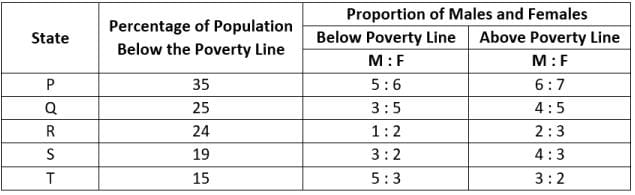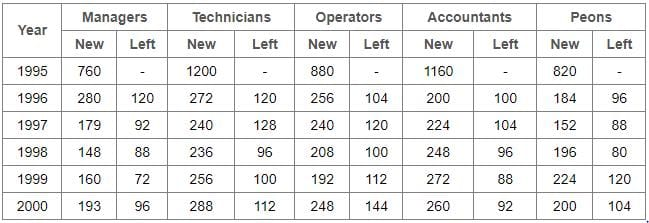Practice Test: Table Charts - 1

# Practice Test: Table Charts - 1

Test Description

## 5 Questions MCQ Test General Aptitude for GATE | Practice Test: Table Charts - 1

Practice Test: Table Charts - 1 for Banking Exams 2023 is part of General Aptitude for GATE preparation. The Practice Test: Table Charts - 1 questions and answers have been prepared according to the Banking Exams exam syllabus.The Practice Test: Table Charts - 1 MCQs are made for Banking Exams 2023 Exam. Find important definitions, questions, notes, meanings, examples, exercises, MCQs and online tests for Practice Test: Table Charts - 1 below.
Solutions of Practice Test: Table Charts - 1 questions in English are available as part of our General Aptitude for GATE for Banking Exams & Practice Test: Table Charts - 1 solutions in Hindi for General Aptitude for GATE course. Download more important topics, notes, lectures and mock test series for Banking Exams Exam by signing up for free. Attempt Practice Test: Table Charts - 1 | 5 questions in 10 minutes | Mock test for Banking Exams preparation | Free important questions MCQ to study General Aptitude for GATE for Banking Exams Exam | Download free PDF with solutions
 1 Crore+ students have signed up on EduRev. Have you?
Practice Test: Table Charts - 1 - Question 1

### The following table gives the percentage distribution of the population of five states, P, Q, R, S and T on the basis of the poverty line and also on the basis of sex.Q. What will be the number of females above the poverty line in the state S if it is known that the population of State S is 7 million?

Detailed Solution for Practice Test: Table Charts - 1 - Question 1

Total population of state S = 7 million
Population above poverty line is:

= [(100 - 19)% of 7] million
= (81% of 7) million
= 5.67 million

So the number of females above the poverty line in the state of S is:

= (3 / 7) * 5.67 million
= 2.43 million

Practice Test: Table Charts - 1 - Question 2

### The following table shows the number of new employees added to different categories of employees in a company and also the number of employees from these categories who left the company every year since the foundation of the Company in 1995.Q. What is the difference between the total number of Technicians added to the Company and the total number of Accountants added to the Company during the years 1996 to 2000?

Detailed Solution for Practice Test: Table Charts - 1 - Question 2

Required difference is:

= (272 + 240 + 236 + 256 + 288) - (200 + 224 + 248 + 272 + 260)
= 88

Practice Test: Table Charts - 1 - Question 3

### The following table gives the percentage distribution of the population of five states, P, Q, R, S and T on the basis of the poverty line and also on the basis of sex.Q. What will be the male population above the poverty line for State P if the female population below the poverty line for State P is 2.1 million?

Detailed Solution for Practice Test: Table Charts - 1 - Question 3

Female population below poverty line for state P = 2.1 million
Let the male population below poverty line for state P be 'x' million.

5 : 6 = x : 21
x = 2.1 * (5 / 6) = 1.75

∴ Population below poverty line for state P = (2.1 + 1.75) million = 3.85 million
Let the population above poverty line for state P be 'y' million
Since, 35% of the total population of state P is below poverty line.
Therefore, 65% of the total population of state P is above poverty line i.e., the ratio of population below poverty line to that above poverty line of state P is 35 : 65

35 : 65 = 3.85 : y
y = 65 * 3.85 / 35 = 7.15

∴ Population above poverty line for state P = 7.15 million and so, male population above poverty line for state P is:

= (6 / 13) * 7.15 million
= 3.3 million

Practice Test: Table Charts - 1 - Question 4

The following table gives the percentage distribution of the population of five states, P, Q, R, S and T on the basis of the poverty line and also on the basis of sex.Q. If the male population above the poverty line for State R is 1.9 million, then the total population of State R is?

Detailed Solution for Practice Test: Table Charts - 1 - Question 4

Let the total population of state R be x million.
Then, the population of state R above poverty line is:

= [(100 - 24)% of x] million
= (76 / 100) * x million

So male populaion of state R above poverty line is:

= [2 / 5 * (76 / 100) * x] million

But, it is given that male population of state R above poverty line = 1.9 million.
∴ (2 / 5) * (76 / 100) * x) = 1.9
⇒ x = 5 * 100 * 1.9 / (76 * 2) = 6.25
∴ Total population of state R = 6.25 million.

Practice Test: Table Charts - 1 - Question 5

The following table gives the percentage distribution of the population of five states, P, Q, R, S and T on the basis of the poverty line and also on the basis of sex.Q. If the population of males below the poverty line for State Q is 2.4 million and that for State T is 6 million, then the total populations of States Q and T are in the ratio?

Detailed Solution for Practice Test: Table Charts - 1 - Question 5

For state Q:
Male population below poverty line = 2.4 million
Let the female population below the poverty line be 'x' million.

3 : 5 = 2.4 : x
x = 5 * 2.4 / 3 = 4

∴ Total population below poverty line = (2.4 + 4) = 6.4 million
If Nq = 6.4 million ⇒ Nq = (6.4 x 100 / 25) million = 25.6 million
For state T:
Male population below poverty line = 6 million
Let the female population below the poverty line be 'y' million.

3 : 5 = 6 : y
y = 3 * 6 / 5 = 3.6

∴ Total population below poverty line = (2.4 + 4) = 6.4 million
If Nt = 6.4 million ⇒ Nt = (9.6 x 100 / 15) million = 64 million
Required ratio is:

= Nq / Nt = 25.6 / 64 = 0.4 = 2 / 5

## General Aptitude for GATE

73 videos|86 docs|108 tests
 Use Code STAYHOME200 and get INR 200 additional OFF Use Coupon Code
Information about Practice Test: Table Charts - 1 Page
In this test you can find the Exam questions for Practice Test: Table Charts - 1 solved & explained in the simplest way possible. Besides giving Questions and answers for Practice Test: Table Charts - 1, EduRev gives you an ample number of Online tests for practice

## General Aptitude for GATE

73 videos|86 docs|108 tests